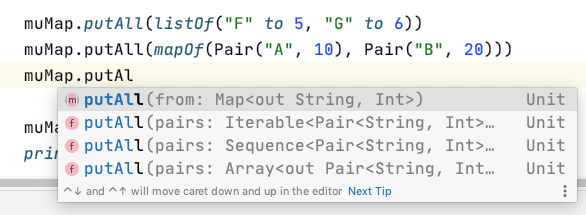## Map

Map 一樣分成

• 只可讀的 Map
• 可以修改的 Mutable Map

• Map 是使用 key, value 來儲存資料的一種資料結構，在其他語言中有時候也被稱為 dictionary (字典)，因為 map 原理就是像查字典一樣，利用關鍵字(key)，找到相對應的資料解釋。
• Map 的存取主要就是透過 key。

## 唯讀的 Map

### mapOf()

``````// to 省略小數點和括號的寫法
val map = mapOf("A" to 10, "B" to 20, "C" to 30) // {A=10, B=20, C=30}

val mapP = mapOf(Pair("A", 10), Pair("B", 20), Pair("C", 30)) // {A=10, B=20, C=30}
``````

## 可以修改的 Mutable Map

### mutableMapOf()

``````mutableMapOf("A" to 10, "B" to 20, "C" to 30)
``````

## 讀取 map

``````println(muMap["A"])
println(muMap.get("A"))
``````

### getValue() - key 不存在時會拋出 NoSuchElementException

``````println(muMap.getValue("D"))
``````

getValue(key) 取不到時會拋出異常

``````Exception in thread "main" java.util.NoSuchElementException: Key D is missing in the map.
at kotlin.collections.MapsKt__MapWithDefaultKt.getOrImplicitDefaultNullable(MapWithDefault.kt:24)
``````

### getOrElse(), getOrDefault()

getOrDefault() 第二個參數則是直接就是找不到就回傳的預設值。

``````println(muMap.getOrElse("E") {"no value"})
println(muMap.getOrDefault("E", 50))
``````

## 新增或修改 map

``````muMap += "D" to 40
muMap["F"] = 100
muMap.put("A", 5)
println("after add or update muMap: \$muMap")
``````

``````after add or update muMap: {A=5, B=20, C=30, D=40, F=100}
``````

### putIfAbsent()

putIfAbsent()，如果 key 存在就不會做更新！

``````muMap.putIfAbsent("A", 100) // "A" 存在所以不更新
``````

### putAll()

putAll() 能放入的方式就比較多種，以下是一些例子### getOrPut()

``````println("getOrPut:" + muMap.getOrPut("G") { 200 })
``````

## 刪除 map

``````muMap.remove("G")
muMap -= "D"
println(muMap)
``````

## loop

``````muMap.forEach {
println(it.key + "," + it.value)
}

muMap.forEach { (key, value) -> println("\$key , \$value") }

for (entry in muMap) {
println(entry.key + "," + entry.value)
}
``````

``````// deconstruct
for ((k,v) in muMap) {
println("\$k,\$v")
}
``````

### HashMap - hashMapOf()

``````hashMapOf("A" to 10, "B" to 20, "C" to 30)
``````

### LinkedHashMap - linkedMapOf()

``````linkedMapOf("A" to 10, "B" to 20, "C" to 30)
``````

### TreeMap - sortedMapOf()

sortedMapOf() 底層是 Java 的 TreeSet，所以會依資料的自然排序做升序排序

``````sortedMapOf("A" to 10, "B" to 20, "C" to 30)
``````

### emptyMap<類型>()

``````emptyMap<String, Int>()
``````

``````// java
println("compute:" + muMap.compute("H") { _: String, _: Int? -> 200 })
println("computeIfAbsent:" + muMap.computeIfAbsent("J") { 100 })
``````

## Leetcode 1. Two Sum

https://leetcode.com/problems/two-sum/

``````fun twoSum(nums: IntArray, target: Int): IntArray {
val map = mutableMapOf<Int, Int>()
nums.forEachIndexed { idx, value ->
if (map.containsKey(target - value)) {
return intArrayOf(map.getValue(target - value), idx)
}
map.put(value, idx)
}
throw RuntimeException("not found")
}
``````

``````fun twoSumSimple(nums: IntArray, target: Int): IntArray {
val map = mutableMapOf<Int, Int>()
nums.forEachIndexed { idx, value ->
map[target - value]?.let { return intArrayOf(it, idx) }
map[value] = idx
}
throw RuntimeException("not found")
}
``````